JMSLTM Numerical Library 7.2.0
com.imsl.math

Class CsPeriodic

• All Implemented Interfaces:
Serializable, Cloneable

public class CsPeriodic
extends Spline
Extension of the Spline class to interpolate data points with periodic boundary conditions.

Class CsPeriodic computes a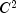cubic spline interpolant to a set of data points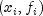for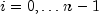. The breakpoints of the spline are the abscissas. The program enforces periodic endpoint conditions. This means that the spline s satisfies s(a) = s(b),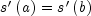, and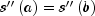, where a is the leftmost abscissa and b is the rightmost abscissa. If the ordinate values corresponding to a and b are not equal, then a warning message is issued. The ordinate value at b is set equal to the ordinate value at a and the interpolant is computed.

If the data points arise from the values of a smooth (say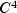) periodic function f, i.e.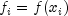, then the error will behave in a predictable fashion. Letbe the breakpoint vector for the above spline interpolant. Then, the maximum absolute error satisfies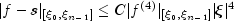where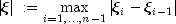For more details, see de Boor (1978, pages 320-322).

Example, Serialized Form
• Constructor Detail

• CsPeriodic

public CsPeriodic(double[] xData,
double[] yData)
Constructs a cubic spline that interpolates the given data points with periodic boundary conditions.
Parameters:
xData - A double array containing the x-coordinates of the data. There must be at least 4 data points and values must be distinct.
yData - A double array containing the y-coordinates of the data. The arrays xData and yData must have the same length.
JMSLTM Numerical Library 7.2.0